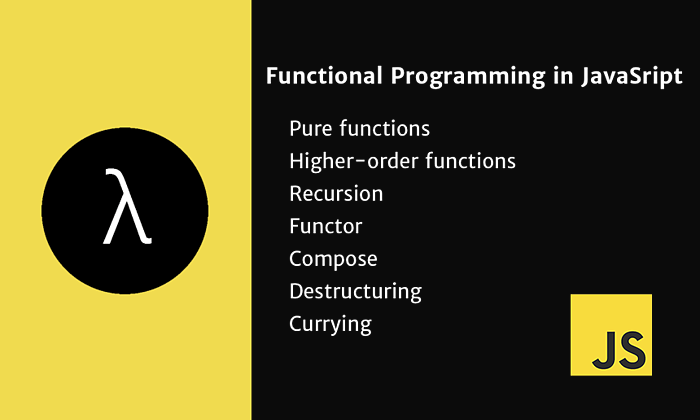# Functional Programming in JavaScript

Tue Sep 20 2016In computer science, functional programming is a programming paradigm—a style of building the structure and elements of computer programs—that treats computation as the evaluation of mathematical functions and avoids changing-state and mutable data. It is a declarative programming paradigm, which means programming is done with expressions or declarations instead of statements. wiki

If you’re looking for a quick start in Functional Programming, JavaScript is the perfect language for you. Here’s why:

• Almost all programmers have tweaked and / or written JavaScript code at some point of time — hence there should be a certain familiarity
• JavaScript comes as close to a standardized programming language we’ll get — it’s the only programming language available across all web browsers
• JavaScript comes with a very familiar `C` like syntax and should be readable to most programmers
• Functions have always been first class members in JavaScript, support for Functional Programming is very good and in many ways JavaScript has been ahead of its time
• JavaScript doesn’t have `Java` like Class based `Object Oriented Programming` support so in many ways you’re forced to be Functional in JavaScript

## Main fancy words you will hear

### Pure functions

Returns the same result given same parameters. It’s execution doesn’t depend on the state of the system.

1. Impure
``````let number = 1
const increment = () => (number += 1)
increment()
// 2
``````
1. Pure
``````const increment = n => n + 1
increment(1)
// 2
``````

### Higher-order functions

Functions that operate on other functions, either by taking them as arguments or by returning them.

1. Sum
``````const sum = (x, y) => x + y
const calculate = (fn, x, y) => fn(x, y)
calculate(sum, 1, 2)
// 3
``````
1. Filter
``````let students = [
{ name: 'Anna', grade: 6 },
{ name: 'John', grade: 4 },
{ name: 'Maria', grade: 9 }
]

const isApproved = student => student.grade >= 6
students.filter(isApproved)
// [ { name: 'Anna', grade: 6 }, { name: 'Maria', grade: 9 } ]
``````
1. Map
``````const byName = obj => obj.name
students.map(byName)
// [ 'Anna', 'John', 'Maria' ]
``````
1. Chaining
``````let students = [
{ name: 'Anna', grade: 6 },
{ name: 'John', grade: 4 },
{ name: 'Maria', grade: 9 }
]

const isApproved = student => student.grade >= 6
const byName = obj => obj.name
students.filter(isApproved).map(byName)
// ['Anna', 'Maria']
``````
1. Reduce
``````const totalGrades = students.reduce((sum, student) => sum + student.grade, 0)
// 19
``````

### Recursion

Whenever a function calls itself, creating a loop.

1. Countdown
``````const countdown = num => {
if (num > 0) {
console.log(num)
countdown(num - 1)
}
}

countdown(5)
/*
5
4
3
2
1
*/
``````
1. Factorial
``````const factorial = num => {
if (num <= 0) {
return 1
} else {
return num * factorial(num - 1)
}
}

factorial(5)
//120
``````

### Functor

An object that has a map method. The map method of the functor takes it’s own contents and transforms each of them using the transformation callback passed to map, and returns a new functor, which contains the structure as the first functor, but with the transformed values.

1. Adding a value to all the elements in a array
``````const plus1 = num => num + 1

let numbers = [1, 2, 3]
numbers.map(plus1)
// [2, 3, 4]
``````

### Compose

The composition of two or more functions returns a new function.

1. Combining two functions to generate another one
``````const compose = (f, g) => x => f(g(x))
const toUpperCase = x => x.toUpperCase()
const exclaim = x => `\${x}!`
const angry = compose(exclaim, toUpperCase)

angry('stop this')
// STOP THIS!
``````
1. Combining three functions to generate another one
``````const compose = (f, g) => x => f(g(x))

const toUpperCase = x => x.toUpperCase()
const exclaim = x => `\${x}!`
const moreExclaim = x => `\${x}!!!!!`
const reallyAngry = compose(exclaim, compose(toUpperCase, moreExclaim))

reallyAngry('stop this')
// STOP THIS!!!!!!
``````

### Destructuring

Extract data from arrays or objects using a syntax that mirrors the construction of array and object literals. Or “Pattern Matching”.

1. Select from pattern
``````const foo = () => [1, 2, 3]

const [a, b] = foo()
console.log(a, b)
// 1 2
``````
1. Accumulates the rest values
``````const [a, ...b] = [1, 2, 3]
console.log(a, b)
// 1 [2, 3]
``````
1. Optional parameters
``````const ajax = ({ url = 'localhost', port: p = 80 }, ...data) =>
console.log('Url:', url, 'Port:', p, 'Rest:', data)

ajax({ url: 'someHost' }, 'additional', 'data', 'hello')
// Url: someHost Port: 80 Rest: [ 'additional', 'data', 'hello' ]

// Url: localhost Port: 80 Rest: [ 'additional', 'data', 'hello' ]
``````

### Currying

Taking a function that takes multiple arguments and turning it into a chain of functions each taking one argument and returning the next function, until the last returns the result.

1. Currying an Object
``````const student = name => grade => `Name: \${name} | Grade: \${grade}`

student('Matt')(8)
// Name: Matt | Grade: 8
``````
1. Currying a Sum
``````const add = x => y => x + y

increment(3)
//4

// 15
``````

#### Sources

https://github.com/js-functional/js-funcional